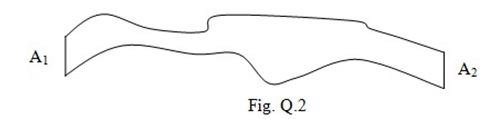# Problems: Conduction - 1D Notes | Study Heat Transfer - Mechanical Engineering

## Mechanical Engineering: Problems: Conduction - 1D Notes | Study Heat Transfer - Mechanical Engineering

The document Problems: Conduction - 1D Notes | Study Heat Transfer - Mechanical Engineering is a part of the Mechanical Engineering Course Heat Transfer.
All you need of Mechanical Engineering at this link: Mechanical Engineering

Problems :  Conduction: One Dimensional, Heat Transfer

Frequently Asked Questions and Problems for Practice

 Q.1 Write the driving force for electricity, fluid, and heat flow and discuss the similarity among them. Q.2 What is the ratio of heat flux through area A1 and area A2 of an irregular pipeline shown in the figure below? The area A1 and A2 are same and the curved surface is well insulated for any kind of heat loss at steady state.Q.3 What is the unit of thermal conductivity? Q.4 What is the significance of log mean area? Q.5 A hollow cylinder has two different layers of insulation of same thickness but different thermal conductivity. The outer diameter of the insulated cylinder is double that of the inner diameter of the cylinder. What will be the change in heat flow if the insulation layers are interchanged considering the same temperature driving force? The thermal conductivity of the inner layer is considered to be the four times that of the other layer for previous case. Q.6 A thick wall of 30 cm thick and 20 W/(m·oC) of thermal conductivity has one surface (maintained at 250oC) and the opposite surface is completely insulated. The heat is generated in the wall at a uniform volumetric rate of 180 kW/m3. Determine the following,a. the temperature distribution in the wall at steady state,b. the maximum wall temperature and its location, andc. the average wall temperature. Q.7 A hollow aluminium sphere having inner diameter of 5 cm and outer diameter of 10 cm is maintained at 100 oC and 50 oC at inside and outside of the sphere. Calculate the heat flux at the outer surface. Q.8 A hot steam pipe (k = 50 W/m·oC) having an inner diameter of 8 cm is at 250 oC. The thickness of the wall is 5.5 mm. The pipe is covered with a 90 mm layer of insulation (k = 0.2 W/m·oC) followed by a 40 mm layer of insulation (k = 0.3 W/m·oC) . The outside temperature of the insulation is 20 oC. Calculate the heat loss per unit of the pipe length. Q.9 Consider a plane wall having uniformly distributed heat sources and one face maintained at a temperature T1 while the other face is maintained at a temperature T2. The thickness of the wall may be taken as 2t. Derive an expression for the temperature distribution in the plane wall. Q.10 Derive an expression for the temperature distribution in a sphere of radius R with uniform heat generation and constant surface temperature.
The document Problems: Conduction - 1D Notes | Study Heat Transfer - Mechanical Engineering is a part of the Mechanical Engineering Course Heat Transfer.
All you need of Mechanical Engineering at this link: Mechanical EngineeringUse Code STAYHOME200 and get INR 200 additional OFF

## Heat Transfer

58 videos|70 docs|85 tests

Track your progress, build streaks, highlight & save important lessons and more!

,

,

,

,

,

,

,

,

,

,

,

,

,

,

,

,

,

,

,

,

,

;